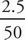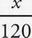# SAT Math Multiple Choice Question 35: Answer and Explanation

### Test Information

Question: 35

5. The amount of time that Amy walks is directly proportional to the distance that she walks. If she walks a distance of 2.5 miles in 50 minutes, how many miles will she walk in 2 hours?

• A. 4.5
• B. 5
• C. 6
• D. 6.5

C Since we know the time that Amy walked and the distance she walked are directly proportional, we can set up a proportion to show her distance ÷ time. We want to know how many miles she'll walk in two hours, so put 120 (60 × 2) minutes in the second half of the ratio:=. To solve, cross-multiply, and you'll get 50x = 2.5 × 120; 50x = 300; x = 6 miles, which is (C).